xLie groupOverview

In mathematics
Mathematics
Mathematics is the study of quantity, space, structure, and change. Mathematicians seek out patterns and formulate new conjectures. Mathematicians resolve the truth or falsity of conjectures by mathematical proofs, which are arguments sufficient to convince other mathematicians of their validity...

, a Lie group (icon) is a group
Group (mathematics)
In mathematics, a group is an algebraic structure consisting of a set together with an operation that combines any two of its elements to form a third element. To qualify as a group, the set and the operation must satisfy a few conditions called group axioms, namely closure, associativity, identity...

which is also a differentiable manifold
Differentiable manifold
A differentiable manifold is a type of manifold that is locally similar enough to a linear space to allow one to do calculus. Any manifold can be described by a collection of charts, also known as an atlas. One may then apply ideas from calculus while working within the individual charts, since...

, with the property that the group operations are compatible with the smooth structure
Differential structure
In mathematics, an n-dimensional differential structure on a set M makes M into an n-dimensional differential manifold, which is a topological manifold with some additional structure that allows us to do differential calculus on the manifold...

. Lie groups are named after Sophus Lie
Sophus Lie
Marius Sophus Lie was a Norwegian mathematician. He largely created the theory of continuous symmetry, and applied it to the study of geometry and differential equations.- Biography :...

, who laid the foundations of the theory of continuous transformation groups.

Lie groups represent the best-developed theory of continuous symmetry
Continuous symmetry
In mathematics, continuous symmetry is an intuitive idea corresponding to the concept of viewing some symmetries as motions, as opposed to e.g. reflection symmetry, which is invariance under a kind of flip from one state to another. It has largely and successfully been formalised in the...

of mathematical object
Mathematical object
In mathematics and the philosophy of mathematics, a mathematical object is an abstract object arising in mathematics.Commonly encountered mathematical objects include numbers, permutations, partitions, matrices, sets, functions, and relations...

s and structures
Mathematical structure
In mathematics, a structure on a set, or more generally a type, consists of additional mathematical objects that in some manner attach to the set, making it easier to visualize or work with, or endowing the collection with meaning or significance....

, which makes them indispensable tools for many parts of contemporary mathematics, as well as for modern theoretical physics
Theoretical physics
Theoretical physics is a branch of physics which employs mathematical models and abstractions of physics to rationalize, explain and predict natural phenomena...

.Unanswered QuestionsWhat is the lie algera of the lie group E(3)?Prove the path connected lie group is connected.Encyclopedia
In mathematics
Mathematics
Mathematics is the study of quantity, space, structure, and change. Mathematicians seek out patterns and formulate new conjectures. Mathematicians resolve the truth or falsity of conjectures by mathematical proofs, which are arguments sufficient to convince other mathematicians of their validity...

, a Lie group (icon) is a group
Group (mathematics)
In mathematics, a group is an algebraic structure consisting of a set together with an operation that combines any two of its elements to form a third element. To qualify as a group, the set and the operation must satisfy a few conditions called group axioms, namely closure, associativity, identity...

which is also a differentiable manifold
Differentiable manifold
A differentiable manifold is a type of manifold that is locally similar enough to a linear space to allow one to do calculus. Any manifold can be described by a collection of charts, also known as an atlas. One may then apply ideas from calculus while working within the individual charts, since...

, with the property that the group operations are compatible with the smooth structure
Differential structure
In mathematics, an n-dimensional differential structure on a set M makes M into an n-dimensional differential manifold, which is a topological manifold with some additional structure that allows us to do differential calculus on the manifold...

. Lie groups are named after Sophus Lie
Sophus Lie
Marius Sophus Lie was a Norwegian mathematician. He largely created the theory of continuous symmetry, and applied it to the study of geometry and differential equations.- Biography :...

, who laid the foundations of the theory of continuous transformation groups.

Lie groups represent the best-developed theory of continuous symmetry
Continuous symmetry
In mathematics, continuous symmetry is an intuitive idea corresponding to the concept of viewing some symmetries as motions, as opposed to e.g. reflection symmetry, which is invariance under a kind of flip from one state to another. It has largely and successfully been formalised in the...

of mathematical object
Mathematical object
In mathematics and the philosophy of mathematics, a mathematical object is an abstract object arising in mathematics.Commonly encountered mathematical objects include numbers, permutations, partitions, matrices, sets, functions, and relations...

s and structures
Mathematical structure
In mathematics, a structure on a set, or more generally a type, consists of additional mathematical objects that in some manner attach to the set, making it easier to visualize or work with, or endowing the collection with meaning or significance....

, which makes them indispensable tools for many parts of contemporary mathematics, as well as for modern theoretical physics
Theoretical physics
Theoretical physics is a branch of physics which employs mathematical models and abstractions of physics to rationalize, explain and predict natural phenomena...

. They provide a natural framework for analysing the continuous symmetries of differential equations (Differential Galois theory
Differential Galois theory
In mathematics, differential Galois theory studies the Galois groups of differential equations.Whereas algebraic Galois theory studies extensions of algebraic fields, differential Galois theory studies extensions of differential fields, i.e. fields that are equipped with a derivation, D. Much of...

), in much the same way as permutation groups are used in Galois theory
Galois theory
In mathematics, more specifically in abstract algebra, Galois theory, named after Évariste Galois, provides a connection between field theory and group theory...

for analysing the discrete symmetries of algebraic equations. An extension of Galois theory to the case of continuous symmetry groups was one of Lie's principal motivations.

## Overview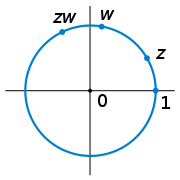Lie groups are smooth manifolds and, therefore, can be studied using differential calculus
Differential calculus
In mathematics, differential calculus is a subfield of calculus concerned with the study of the rates at which quantities change. It is one of the two traditional divisions of calculus, the other being integral calculus....

, in contrast with the case of more general topological group
Topological group
In mathematics, a topological group is a group G together with a topology on G such that the group's binary operation and the group's inverse function are continuous functions with respect to the topology. A topological group is a mathematical object with both an algebraic structure and a...

s. One of the key ideas in the theory of Lie groups is to replace the global object, the group, with its local or linearized version, which Lie himself called its "infinitesimal group" and which has since become known as its Lie algebra
Lie algebra
In mathematics, a Lie algebra is an algebraic structure whose main use is in studying geometric objects such as Lie groups and differentiable manifolds. Lie algebras were introduced to study the concept of infinitesimal transformations. The term "Lie algebra" was introduced by Hermann Weyl in the...

.

Lie groups play an enormous role in modern geometry
Geometry
Geometry arose as the field of knowledge dealing with spatial relationships. Geometry was one of the two fields of pre-modern mathematics, the other being the study of numbers ....

, on several different levels. Felix Klein
Felix Klein
Christian Felix Klein was a German mathematician, known for his work in group theory, function theory, non-Euclidean geometry, and on the connections between geometry and group theory...

argued in his Erlangen program
Erlangen program
An influential research program and manifesto was published in 1872 by Felix Klein, under the title Vergleichende Betrachtungen über neuere geometrische Forschungen...

that one can consider various "geometries" by specifying an appropriate transformation group that leaves certain geometric properties invariant. Thus Euclidean geometry
Euclidean geometry
Euclidean geometry is a mathematical system attributed to the Alexandrian Greek mathematician Euclid, which he described in his textbook on geometry: the Elements. Euclid's method consists in assuming a small set of intuitively appealing axioms, and deducing many other propositions from these...

corresponds to the choice of the group E(3)
Euclidean group
In mathematics, the Euclidean group E, sometimes called ISO or similar, is the symmetry group of n-dimensional Euclidean space...

of distance-preserving transformations of the Euclidean space R3, conformal geometry
Conformal geometry
In mathematics, conformal geometry is the study of the set of angle-preserving transformations on a space. In two real dimensions, conformal geometry is precisely the geometry of Riemann surfaces...

corresponds to enlarging the group to the conformal group
Conformal group
In mathematics, the conformal group is the group of transformations from a space to itself that preserve all angles within the space. More formally, it is the group of transformations that preserve the conformal geometry of the space. Several specific conformal groups are particularly important:*...

, whereas in projective geometry
Projective geometry
In mathematics, projective geometry is the study of geometric properties that are invariant under projective transformations. This means that, compared to elementary geometry, projective geometry has a different setting, projective space, and a selective set of basic geometric concepts...

one is interested in the properties invariant under the projective group. This idea later led to the notion of a G-structure
G-structure
In differential geometry, a G-structure on an n-manifold M, for a given structure group G, is a G-subbundle of the tangent frame bundle FM of M....

, where G is a Lie group of "local" symmetries of a manifold. On a "global" level, whenever a Lie group acts
Group action
In algebra and geometry, a group action is a way of describing symmetries of objects using groups. The essential elements of the object are described by a set, and the symmetries of the object are described by the symmetry group of this set, which consists of bijective transformations of the set...

on a geometric object, such as a Riemannian
Riemannian manifold
In Riemannian geometry and the differential geometry of surfaces, a Riemannian manifold or Riemannian space is a real differentiable manifold M in which each tangent space is equipped with an inner product g, a Riemannian metric, which varies smoothly from point to point...

or a symplectic manifold
Symplectic manifold
In mathematics, a symplectic manifold is a smooth manifold, M, equipped with a closed nondegenerate differential 2-form, ω, called the symplectic form. The study of symplectic manifolds is called symplectic geometry or symplectic topology...

, this action provides a measure of rigidity and yields a rich algebraic structure. The presence of continuous symmetries expressed via a Lie group action on a manifold places strong constraints on its geometry and facilitates analysis on the manifold. Linear actions of Lie groups are especially important, and are studied in representation theory
Representation theory
Representation theory is a branch of mathematics that studies abstract algebraic structures by representing their elements as linear transformations of vector spaces, and studiesmodules over these abstract algebraic structures...

.

In the 1940s–1950s, Ellis Kolchin, Armand Borel and Claude Chevalley realised that many foundational results concerning Lie groups can be developed completely algebraically, giving rise to the theory of algebraic group
Algebraic group
In algebraic geometry, an algebraic group is a group that is an algebraic variety, such that the multiplication and inverse are given by regular functions on the variety...

s defined over an arbitrary field
Field (mathematics)
In abstract algebra, a field is a commutative ring whose nonzero elements form a group under multiplication. As such it is an algebraic structure with notions of addition, subtraction, multiplication, and division, satisfying certain axioms...

. This insight opened new possibilities in pure algebra, by providing a uniform construction for most finite simple groups, as well as in algebraic geometry
Algebraic geometry
Algebraic geometry is a branch of mathematics which combines techniques of abstract algebra, especially commutative algebra, with the language and the problems of geometry. It occupies a central place in modern mathematics and has multiple conceptual connections with such diverse fields as complex...

. The theory of automorphic form
Automorphic form
In mathematics, the general notion of automorphic form is the extension to analytic functions, perhaps of several complex variables, of the theory of modular forms...

s, an important branch of modern number theory
Number theory
Number theory is a branch of pure mathematics devoted primarily to the study of the integers. Number theorists study prime numbers as well...

, deals extensively with analogues of Lie groups over adele ring
Adele ring
In algebraic number theory and topological algebra, the adele ring is a topological ring which is built on the field of rational numbers . It involves all the completions of the field....

s; p-adic Lie groups play an important role, via their connections with Galois representations in number theory.

## Definitions and examples

A real Lie group is a group
Group (mathematics)
In mathematics, a group is an algebraic structure consisting of a set together with an operation that combines any two of its elements to form a third element. To qualify as a group, the set and the operation must satisfy a few conditions called group axioms, namely closure, associativity, identity...

which is also a finite-dimensional real smooth manifold, and in which the group operations of multiplication
Multiplication
Multiplication is the mathematical operation of scaling one number by another. It is one of the four basic operations in elementary arithmetic ....

and inversion are smooth maps. Smoothness of the group multiplication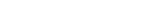means that μ is a smooth mapping of the product manifold G×G into G. These two requirements can be combined to the single requirement that the mapping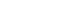be a smooth mapping of the product manifold into G.

### First examples

• The 2×2 real
Real number
In mathematics, a real number is a value that represents a quantity along a continuum, such as -5 , 4/3 , 8.6 , √2 and π...

invertible matrices form a group under multiplication, denoted by GL2(R):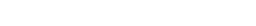This is a four-dimensional noncompact
Compact space
In mathematics, specifically general topology and metric topology, a compact space is an abstract mathematical space whose topology has the compactness property, which has many important implications not valid in general spaces...

real Lie group. This group is disconnected
Connected space
In topology and related branches of mathematics, a connected space is a topological space that cannot be represented as the union of two or more disjoint nonempty open subsets. Connectedness is one of the principal topological properties that is used to distinguish topological spaces...

; it has two connected components corresponding to the positive and negative values of the determinant
Determinant
In linear algebra, the determinant is a value associated with a square matrix. It can be computed from the entries of the matrix by a specific arithmetic expression, while other ways to determine its value exist as well...

.

• The rotation
Rotation (mathematics)
In geometry and linear algebra, a rotation is a transformation in a plane or in space that describes the motion of a rigid body around a fixed point. A rotation is different from a translation, which has no fixed points, and from a reflection, which "flips" the bodies it is transforming...

matrices form a subgroup
Subgroup
In group theory, given a group G under a binary operation *, a subset H of G is called a subgroup of G if H also forms a group under the operation *. More precisely, H is a subgroup of G if the restriction of * to H x H is a group operation on H...

of GL2(R), denoted by SO2(R). It is a Lie group in its own right: specifically, a one-dimensional compact connected Lie group which is diffeomorphic to the circle
Circle
A circle is a simple shape of Euclidean geometry consisting of those points in a plane that are a given distance from a given point, the centre. The distance between any of the points and the centre is called the radius....

. Using the rotation angle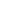as a parameter, this group can be parametrized as follows: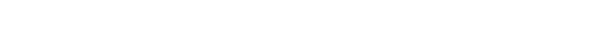Addition of the angles corresponds to multiplication of the elements of SO2(R), and taking the opposite angle corresponds to inversion. Thus both multiplication and inversion are differentiable maps.

• The orthogonal group
Orthogonal group
In mathematics, the orthogonal group of degree n over a field F is the group of n × n orthogonal matrices with entries from F, with the group operation of matrix multiplication...

also forms an interesting example of a Lie group.

All of the previous examples of Lie groups fall within the class of classical group
Classical group
In mathematics, the classical Lie groups are four infinite families of Lie groups closely related to the symmetries of Euclidean spaces. Their finite analogues are the classical groups of Lie type...

s

### Analytic subgroup

An analytic subgroup H of a Lie group G is a subgroup which is a connected Lie group, and for which the inclusion mapping is smooth
Smooth
Smooth means having a texture that lacks friction. Not rough.Smooth may also refer to:-In mathematics:* Smooth function, a function that is infinitely differentiable; used in calculus and topology...

and everywhere regular. Note that the analytic subgroup H is itself an analytic group.

Let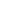and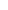be the Lie algebras of H and G, and let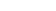be the inclusion map. Then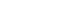is an injective Lie algebra
Lie algebra
In mathematics, a Lie algebra is an algebraic structure whose main use is in studying geometric objects such as Lie groups and differentiable manifolds. Lie algebras were introduced to study the concept of infinitesimal transformations. The term "Lie algebra" was introduced by Hermann Weyl in the...

homomorphism
Homomorphism
In abstract algebra, a homomorphism is a structure-preserving map between two algebraic structures . The word homomorphism comes from the Greek language: ὁμός meaning "same" and μορφή meaning "shape".- Definition :The definition of homomorphism depends on the type of algebraic structure under...

: any analytic subgroup gives a Lie subalgebra in a natural way. This can be seen as a bijective map from analytic subgroups of G to Lie subalgebras of.

### Related concepts

A complex Lie group is defined in the same way using complex manifold
Complex manifold
In differential geometry, a complex manifold is a manifold with an atlas of charts to the open unit disk in Cn, such that the transition maps are holomorphic....

s rather than real ones (example: SL2(C)), and similarly one can define a p-adic Lie group over the p-adic numbers
P-adic number
In mathematics, and chiefly number theory, the p-adic number system for any prime number p extends the ordinary arithmetic of the rational numbers in a way different from the extension of the rational number system to the real and complex number systems...

. Hilbert's fifth problem
Hilbert's fifth problem
Hilbert's fifth problem, is the fifth mathematical problem from the problem-list publicized in 1900 by mathematician David Hilbert, and concerns the characterization of Lie groups. The theory of Lie groups describes continuous symmetry in mathematics; its importance there and in theoretical physics...

asked whether replacing differentiable manifolds with topological or analytic ones can yield new examples. The answer to this question turned out to be negative: in 1952, Gleason
Andrew Gleason
Andrew Mattei Gleason was an American mathematician and the eponym of Gleason's theorem and the Greenwood–Gleason graph. After briefly attending Berkeley High School he graduated from Roosevelt High School in Yonkers, then Yale University in 1942, where he became a Putnam Fellow...

, Montgomery
Deane Montgomery
Deane Montgomery was a mathematician specializing in topology who was one of the contributors to the final resolution of Hilbert's fifth problem in the 1950s. He served as President of the American Mathematical Society from 1961 to 1962....

and Zippin
Leo Zippin
Leo Zippin was an American mathematician. His parents were Bella Salwen and Max Zippin who immigrated to New York City from the Ukraine in 1903.-Education:...

showed that if G is a topological manifold with continuous group operations, then there exists exactly one analytic structure on G which turns it into a Lie group (see also Hilbert–Smith conjecture). If the underlying manifold is allowed to be infinite dimensional (for example, a Hilbert manifold
Hilbert manifold
In mathematics, a Hilbert manifold is a manifold modeled on Hilbert spaces. Thus it is a separable Hausdorff space in which each point has a neighbourhood homeomorphic to an infinite dimensional Hilbert space. The concept of a Hilbert manifold provides a possibility of extending the theory of...

) then one arrives at the notion of an infinite-dimensional Lie group. It is possible to define analogues of many Lie groups over finite fields
Group of Lie type
In mathematics, a group of Lie type G is a group of rational points of a reductive linear algebraic group G with values in the field k. Finite groups of Lie type form the bulk of nonabelian finite simple groups...

, and these give most of the examples of finite simple groups.

The language of category theory
Category theory
Category theory is an area of study in mathematics that examines in an abstract way the properties of particular mathematical concepts, by formalising them as collections of objects and arrows , where these collections satisfy certain basic conditions...

provides a concise definition for Lie groups: a Lie group is a group object
Group object
In category theory, a branch of mathematics, group objects are certain generalizations of groups which are built on more complicated structures than sets...

in the category
Category (mathematics)
In mathematics, a category is an algebraic structure that comprises "objects" that are linked by "arrows". A category has two basic properties: the ability to compose the arrows associatively and the existence of an identity arrow for each object. A simple example is the category of sets, whose...

of smooth manifolds. This is important, because it allows generalization of the notion of a Lie group to Lie supergroups
Supergroup (physics)
The concept of supergroup is a generalization of that of group. In other words, every group is a supergroup but not every supergroup is a group. A supergroup is like a Lie group in that there is a well defined notion of smooth function defined on them....

.

## More examples of Lie groups

Lie groups occur in abundance throughout mathematics and physics. Matrix group
Matrix group
In mathematics, a matrix group is a group G consisting of invertible matrices over some field K, usually fixed in advance, with operations of matrix multiplication and inversion. More generally, one can consider n × n matrices over a commutative ring R...

s or algebraic group
Algebraic group
In algebraic geometry, an algebraic group is a group that is an algebraic variety, such that the multiplication and inverse are given by regular functions on the variety...

s are (roughly) groups of matrices (for example, orthogonal
Orthogonal group
In mathematics, the orthogonal group of degree n over a field F is the group of n × n orthogonal matrices with entries from F, with the group operation of matrix multiplication...

and symplectic group
Symplectic group
In mathematics, the name symplectic group can refer to two different, but closely related, types of mathematical groups, denoted Sp and Sp. The latter is sometimes called the compact symplectic group to distinguish it from the former. Many authors prefer slightly different notations, usually...

s), and these give most of the more common examples of Lie groups.

### Examples

• Euclidean space
Euclidean space
In mathematics, Euclidean space is the Euclidean plane and three-dimensional space of Euclidean geometry, as well as the generalizations of these notions to higher dimensions...

Rn with ordinary vector addition as the group operation becomes an n-dimensional noncompact abelian
Abelian group
In abstract algebra, an abelian group, also called a commutative group, is a group in which the result of applying the group operation to two group elements does not depend on their order . Abelian groups generalize the arithmetic of addition of integers...

Lie group.
• The circle group S1 consisting of angles mod 2π under addition or, alternately, the complex numbers with absolute value 1 under multiplication. This is a one-dimensional compact connected abelian Lie group.
• The group GLn(R) of invertible matrices
Matrix (mathematics)
In mathematics, a matrix is a rectangular array of numbers, symbols, or expressions. The individual items in a matrix are called its elements or entries. An example of a matrix with six elements isMatrices of the same size can be added or subtracted element by element...

(under matrix multiplication
Matrix multiplication
In mathematics, matrix multiplication is a binary operation that takes a pair of matrices, and produces another matrix. If A is an n-by-m matrix and B is an m-by-p matrix, the result AB of their multiplication is an n-by-p matrix defined only if the number of columns m of the left matrix A is the...

) is a Lie group of dimension n2, called the general linear group
General linear group
In mathematics, the general linear group of degree n is the set of n×n invertible matrices, together with the operation of ordinary matrix multiplication. This forms a group, because the product of two invertible matrices is again invertible, and the inverse of an invertible matrix is invertible...

. It has a closed connected subgroup SLn(R), the special linear group
Special linear group
In mathematics, the special linear group of degree n over a field F is the set of n×n matrices with determinant 1, with the group operations of ordinary matrix multiplication and matrix inversion....

, consisting of matrices of determinant 1 which is also a Lie group.
• The orthogonal group
Orthogonal group
In mathematics, the orthogonal group of degree n over a field F is the group of n × n orthogonal matrices with entries from F, with the group operation of matrix multiplication...

On(R), consisting of all n × n orthogonal matrices
Orthogonal matrix
In linear algebra, an orthogonal matrix , is a square matrix with real entries whose columns and rows are orthogonal unit vectors ....

with real entries is an n(n − 1)/2-dimensional Lie group. This group is disconnected, but it has a connected subgroup SOn(R) of the same dimension consisting of orthogonal matrices of determinant 1, called the special orthogonal group (for n = 3, the rotation group
Rotation group
In mechanics and geometry, the rotation group is the group of all rotations about the origin of three-dimensional Euclidean space R3 under the operation of composition. By definition, a rotation about the origin is a linear transformation that preserves length of vectors and preserves orientation ...

).
• The Euclidean group
Euclidean group
In mathematics, the Euclidean group E, sometimes called ISO or similar, is the symmetry group of n-dimensional Euclidean space...

En(R) is the Lie group of all Euclidean motions, i.e., isometric affine maps, of n-dimensional Euclidean space Rn.
• The unitary group
Unitary group
In mathematics, the unitary group of degree n, denoted U, is the group of n×n unitary matrices, with the group operation that of matrix multiplication. The unitary group is a subgroup of the general linear group GL...

U(n) consisting of n × n unitary matrices (with complex entries) is a compact connected Lie group of dimension n2. Unitary matrices of determinant 1 form a closed connected subgroup of dimension n2 − 1 denoted SU(n), the special unitary group
Special unitary group
The special unitary group of degree n, denoted SU, is the group of n×n unitary matrices with determinant 1. The group operation is that of matrix multiplication...

.
• Spin groups are double covers of the special orthogonal groups, used for studying fermion
Fermion
In particle physics, a fermion is any particle which obeys the Fermi–Dirac statistics . Fermions contrast with bosons which obey Bose–Einstein statistics....

s in quantum field theory
Quantum field theory
Quantum field theory provides a theoretical framework for constructing quantum mechanical models of systems classically parametrized by an infinite number of dynamical degrees of freedom, that is, fields and many-body systems. It is the natural and quantitative language of particle physics and...

(among other things).
• The symplectic group
Symplectic group
In mathematics, the name symplectic group can refer to two different, but closely related, types of mathematical groups, denoted Sp and Sp. The latter is sometimes called the compact symplectic group to distinguish it from the former. Many authors prefer slightly different notations, usually...

Sp2n(R) consists of all 2n × 2n matrices preserving a nondegenerate skew-symmetric bilinear form on R2n (the symplectic form). It is a connected Lie group of dimension 2n2 + n. The fundamental group
Fundamental group
In mathematics, more specifically algebraic topology, the fundamental group is a group associated to any given pointed topological space that provides a way of determining when two paths, starting and ending at a fixed base point, can be continuously deformed into each other...

of the symplectic group is Z and this fact is related to the theory of Maslov index.
• The 3-sphere
3-sphere
In mathematics, a 3-sphere is a higher-dimensional analogue of a sphere. It consists of the set of points equidistant from a fixed central point in 4-dimensional Euclidean space...

S3 forms a Lie group by identification with the set of quaternion
Quaternion
In mathematics, the quaternions are a number system that extends the complex numbers. They were first described by Irish mathematician Sir William Rowan Hamilton in 1843 and applied to mechanics in three-dimensional space...

s of unit norm, called versors. The only other spheres that admit the structure of a Lie group are the 0-sphere S0 (real numbers with absolute value 1) and the circle S1 (complex numbers with absolute value 1). For example, for even n > 1, Sn is not a Lie group because it does not admit a nonvanishing vector field and so link a fortiori
A fortiori argument
The Latin phrase ' denotes "argument 'from [the] stronger [reason]'." For example, if it has been established that a person is deceased, then one can, with equal or greater certainty, argue that the person is not breathing.-Usage:...

cannot be parallelizable as a differentiable manifold. Of the spheres only S0, S1, S3, and S7 are parallelizable. The latter carries the structure of a Lie quasigroup
Quasigroup
In mathematics, especially in abstract algebra, a quasigroup is an algebraic structure resembling a group in the sense that "division" is always possible...

(a nonassociative group), which can be identified with the set of unit octonions.
• The group of upper triangular n by n matrices is a solvable Lie group
Solvable group
In mathematics, more specifically in the field of group theory, a solvable group is a group that can be constructed from abelian groups using extensions...

of dimension n(n + 1)/2.
• The Lorentz group
Lorentz group
In physics , the Lorentz group is the group of all Lorentz transformations of Minkowski spacetime, the classical setting for all physical phenomena...

and the Poincaré group
Poincaré group
In physics and mathematics, the Poincaré group, named after Henri Poincaré, is the group of isometries of Minkowski spacetime.-Simple explanation:...

are the groups of linear and affine isometries
Isometry
In mathematics, an isometry is a distance-preserving map between metric spaces. Geometric figures which can be related by an isometry are called congruent.Isometries are often used in constructions where one space is embedded in another space...

of the Minkowski space
Minkowski space
In physics and mathematics, Minkowski space or Minkowski spacetime is the mathematical setting in which Einstein's theory of special relativity is most conveniently formulated...

(interpreted as the spacetime
Spacetime
In physics, spacetime is any mathematical model that combines space and time into a single continuum. Spacetime is usually interpreted with space as being three-dimensional and time playing the role of a fourth dimension that is of a different sort from the spatial dimensions...

of the special relativity
Special relativity
Special relativity is the physical theory of measurement in an inertial frame of reference proposed in 1905 by Albert Einstein in the paper "On the Electrodynamics of Moving Bodies".It generalizes Galileo's...

). They are Lie groups of dimensions 6 and 10.
• The Heisenberg group is a connected nilpotent
Nilpotent group
In mathematics, more specifically in the field of group theory, a nilpotent group is a group that is "almost abelian". This idea is motivated by the fact that nilpotent groups are solvable, and for finite nilpotent groups, two elements having relatively prime orders must commute...

Lie group of dimension 3, playing a key role in quantum mechanics
Quantum mechanics
Quantum mechanics, also known as quantum physics or quantum theory, is a branch of physics providing a mathematical description of much of the dual particle-like and wave-like behavior and interactions of energy and matter. It departs from classical mechanics primarily at the atomic and subatomic...

.
• The group U(1)×SU(2)×SU(3) is a Lie group of dimension 1+3+8=12 that is the gauge group of the Standard Model
Standard Model
The Standard Model of particle physics is a theory concerning the electromagnetic, weak, and strong nuclear interactions, which mediate the dynamics of the known subatomic particles. Developed throughout the mid to late 20th century, the current formulation was finalized in the mid 1970s upon...

in particle physics
Particle physics
Particle physics is a branch of physics that studies the existence and interactions of particles that are the constituents of what is usually referred to as matter or radiation. In current understanding, particles are excitations of quantum fields and interact following their dynamics...

. The dimensions of the factors correspond to the 1 photon
Photon
In physics, a photon is an elementary particle, the quantum of the electromagnetic interaction and the basic unit of light and all other forms of electromagnetic radiation. It is also the force carrier for the electromagnetic force...

+ 3 vector boson
Vector boson
In particle physics, a vector boson is a boson with the spin quantum number equal to 1.The vector bosons considered to be elementary particles in the Standard Model are the gauge bosons or, the force carriers of fundamental interactions: the photon of electromagnetism, the W and Z bosons of the...

s + 8 gluon
Gluon
Gluons are elementary particles which act as the exchange particles for the color force between quarks, analogous to the exchange of photons in the electromagnetic force between two charged particles....

s of the standard model.
• The (3-dimensional) metaplectic group
Metaplectic group
In mathematics, the metaplectic group Mp2n is a double cover of the symplectic group Sp2n. It can be defined over either real or p-adic numbers...

is a double cover of SL2(R) playing an important role in the theory of modular form
Modular form
In mathematics, a modular form is a analytic function on the upper half-plane satisfying a certain kind of functional equation and growth condition. The theory of modular forms therefore belongs to complex analysis but the main importance of the theory has traditionally been in its connections...

s. It is a connected Lie group that cannot be faithfully represented by matrices of finite size, i.e., a nonlinear group.
• The exceptional Lie groups of types G2, F4, E6, E7, E8
E8 (mathematics)
In mathematics, E8 is any of several closely related exceptional simple Lie groups, linear algebraic groups or Lie algebras of dimension 248; the same notation is used for the corresponding root lattice, which has rank 8...

have dimensions 14, 52, 78, 133, and 248. There is also a group E
E7½ (Lie algebra)
In mathematics, the Lie algebra E7½ is a subalgebra of E8 containing E7 defined by Landsberg and Manivel in orderto fill the "hole" in a dimension formula for the exceptional series En of simple Lie algebras. This hole was observed by Cvitanovic, Deligne, Cohen and de Man...

of dimension 190.

### Constructions

There are several standard ways to form new Lie groups from old ones:
• The product of two Lie groups is a Lie group.
• Any topologically closed
Closed set
In geometry, topology, and related branches of mathematics, a closed set is a set whose complement is an open set. In a topological space, a closed set can be defined as a set which contains all its limit points...

subgroup of a Lie group is a Lie group. This is known as Cartan's theorem
Cartan's theorem
In mathematics, three results in Lie group theory are called Cartan's theorem, named after Élie Cartan:See also Cartan's theorems A and B, results of Henri Cartan, and Cartan's lemma for various other results attributed to Élie and Henri Cartan....

.
• The quotient of a Lie group by a closed normal subgroup is a Lie group.
• The universal cover of a connected Lie group is a Lie group. For example, the group R is the universal cover of the circle group S1. In fact any covering of a differentiable manifold is also a differentiable manifold, but by specifying universal cover, one guarantees a group structure (compatible with its other structures).

### Related notions

Some examples of groups that are not Lie groups (except in the trivial sense that any group can be viewed as a 0-dimensional Lie group, with the discrete topology), are:
• Infinite dimensional groups, such as the additive group of an infinite dimensional real vector space. These are not Lie groups as they are not finite dimensional manifolds

• Some totally disconnected group
Totally disconnected group
In mathematics, a totally disconnected group is a topological group that is totally disconnected. Such topological groups are necessarily Hausdorff....

s, such as the Galois group
Galois group
In mathematics, more specifically in the area of modern algebra known as Galois theory, the Galois group of a certain type of field extension is a specific group associated with the field extension...

of an infinite extension of fields, or the additive group of the p-adic numbers
P-adic number
In mathematics, and chiefly number theory, the p-adic number system for any prime number p extends the ordinary arithmetic of the rational numbers in a way different from the extension of the rational number system to the real and complex number systems...

. These are not Lie groups because their underlying spaces are not real manifolds. (Some of these groups are "p-adic Lie groups"). In general, only topological groups having similar local properties
Local property
In mathematics, a phenomenon is sometimes said to occur locally if, roughly speaking, it occurs on sufficiently small or arbitrarily small neighborhoods of points.-Properties of a single space:...

to Rn for some positive integer n can be Lie groups (of course they must also have a differentiable structure)

## Early history

According to the most authoritative source on the early history of Lie groups (Hawkins, p. 1), Sophus Lie
Sophus Lie
Marius Sophus Lie was a Norwegian mathematician. He largely created the theory of continuous symmetry, and applied it to the study of geometry and differential equations.- Biography :...

himself considered the winter of 1873–1874 as the birth date of his theory of continuous groups. Hawkins, however, suggests that it was "Lie's prodigious research activity during the four-year period from the fall of 1869 to the fall of 1873" that led to the theory's creation (ibid). Some of Lie's early ideas were developed in close collaboration with Felix Klein
Felix Klein
Christian Felix Klein was a German mathematician, known for his work in group theory, function theory, non-Euclidean geometry, and on the connections between geometry and group theory...

. Lie met with Klein every day from October 1869 through 1872: in Berlin from the end of October 1869 to the end of February 1870, and in Paris, Göttingen and Erlangen in the subsequent two years (ibid, p. 2). Lie stated that all of the principal results were obtained by 1884. But during the 1870s all his papers (except the very first note) were published in Norwegian journals, which impeded recognition of the work throughout the rest of Europe (ibid, p. 76). In 1884 a young German mathematician, Friedrich Engel
Friedrich Engel (mathematician)
Friedrich Engel was a German mathematician.Engel was born in Lugau, Saxony, as the son of a Lutheran pastor. He attended the Universities of both Leipzig and Berlin, before receiving his doctorate from Leipzig in 1883.Engel studied under Felix Klein at Leipzig, and collaborated with Sophus Lie for...

, came to work with Lie on a systematic treatise to expose his theory of continuous groups. From this effort resulted the three-volume Theorie der Transformationsgruppen, published in 1888, 1890, and 1893.

Lie's ideas did not stand in isolation from the rest of mathematics. In fact, his interest in the geometry of differential equations was first motivated by the work of Carl Gustav Jacobi, on the theory of partial differential equation
Partial differential equation
In mathematics, partial differential equations are a type of differential equation, i.e., a relation involving an unknown function of several independent variables and their partial derivatives with respect to those variables...

s of first order and on the equations of classical mechanics
Classical mechanics
In physics, classical mechanics is one of the two major sub-fields of mechanics, which is concerned with the set of physical laws describing the motion of bodies under the action of a system of forces...

. Much of Jacobi's work was published posthumously in the 1860s, generating enormous interest in France and Germany (Hawkins, p. 43). Lie's idée fixe was to develop a theory of symmetries of differential equations that would accomplish for them what Évariste Galois
Évariste Galois
Évariste Galois was a French mathematician born in Bourg-la-Reine. While still in his teens, he was able to determine a necessary and sufficient condition for a polynomial to be solvable by radicals, thereby solving a long-standing problem...

had done for algebraic equations: namely, to classify them in terms of group theory. Lie and other mathematicians showed that the most important equations for special functions
Special functions
Special functions are particular mathematical functions which have more or less established names and notations due to their importance in mathematical analysis, functional analysis, physics, or other applications....

and orthogonal polynomials
Orthogonal polynomials
In mathematics, the classical orthogonal polynomials are the most widely used orthogonal polynomials, and consist of the Hermite polynomials, the Laguerre polynomials, the Jacobi polynomials together with their special cases the ultraspherical polynomials, the Chebyshev polynomials, and the...

tend to arise from group theoretical symmetries. Additional impetus to consider continuous groups came from ideas of Bernhard Riemann
Bernhard Riemann
Georg Friedrich Bernhard Riemann was an influential German mathematician who made lasting contributions to analysis and differential geometry, some of them enabling the later development of general relativity....

, on the foundations of geometry, and their further development in the hands of Klein. Thus three major themes in 19th century mathematics were combined by Lie in creating his new theory: the idea of symmetry, as exemplified by Galois through the algebraic notion of a group
Group (mathematics)
In mathematics, a group is an algebraic structure consisting of a set together with an operation that combines any two of its elements to form a third element. To qualify as a group, the set and the operation must satisfy a few conditions called group axioms, namely closure, associativity, identity...

; geometric theory and the explicit solutions of differential equation
Differential equation
A differential equation is a mathematical equation for an unknown function of one or several variables that relates the values of the function itself and its derivatives of various orders...

s of mechanics, worked out by Poisson and Jacobi; and the new understanding of geometry
Geometry
Geometry arose as the field of knowledge dealing with spatial relationships. Geometry was one of the two fields of pre-modern mathematics, the other being the study of numbers ....

that emerged in the works of Plücker
Julius Plücker
Julius Plücker was a German mathematician and physicist. He made fundamental contributions to the field of analytical geometry and was a pioneer in the investigations of cathode rays that led eventually to the discovery of the electron. He also vastly extended the study of Lamé curves.- Early...

, Möbius
August Ferdinand Möbius
August Ferdinand Möbius was a German mathematician and theoretical astronomer.He is best known for his discovery of the Möbius strip, a non-orientable two-dimensional surface with only one side when embedded in three-dimensional Euclidean space. It was independently discovered by Johann Benedict...

, Grassmann and others, and culminated in Riemann's revolutionary vision of the subject.

Although today Sophus Lie is rightfully recognized as the creator of the theory of continuous groups, a major stride in the development of their structure theory, which was to have a profound influence on subsequent development of mathematics, was made by Wilhelm Killing
Wilhelm Killing
Wilhelm Karl Joseph Killing was a German mathematician who made important contributions to the theories of Lie algebras, Lie groups, and non-Euclidean geometry....

, who in 1888 published the first paper in a series entitled
Die Zusammensetzung der stetigen endlichen Transformationsgruppen (The composition of continuous finite transformation groups) (Hawkins, p. 100). The work of Killing, later refined and generalized by Élie Cartan
Élie Cartan
Élie Joseph Cartan was an influential French mathematician, who did fundamental work in the theory of Lie groups and their geometric applications...

, led to classification of semisimple Lie algebras, Cartan's theory of symmetric spaces
Riemannian symmetric space
In differential geometry, representation theory and harmonic analysis, a symmetric space is a smooth manifold whose group of symmetries contains an inversion symmetry about every point. There are two ways to formulate the inversion symmetry, via Riemannian geometry or via Lie theory...

, and Hermann Weyl
Hermann Weyl
Hermann Klaus Hugo Weyl was a German mathematician and theoretical physicist. Although much of his working life was spent in Zürich, Switzerland and then Princeton, he is associated with the University of Göttingen tradition of mathematics, represented by David Hilbert and Hermann Minkowski.His...

's description of representations
Group representation
In the mathematical field of representation theory, group representations describe abstract groups in terms of linear transformations of vector spaces; in particular, they can be used to represent group elements as matrices so that the group operation can be represented by matrix multiplication...

of compact and semisimple Lie groups using highest weights.

Weyl brought the early period of the development of the theory of Lie groups to fruition, for not only did he classify irreducible representations of semisimple Lie groups and connect the theory of groups with quantum mechanics, but he also put Lie's theory itself on firmer footing by clearly enunciating the distinction between Lie's infinitesimal groups (i.e., Lie algebras) and the Lie groups proper, and began investigations of topology of Lie groups (Borel (2001)). The theory of Lie groups was systematically reworked in modern mathematical language in a monograph by Claude Chevalley
Claude Chevalley
Claude Chevalley was a French mathematician who made important contributions to number theory, algebraic geometry, class field theory, finite group theory, and the theory of algebraic groups...

.

## The concept of a Lie group, and possibilities of classification

Lie groups may be thought of as smoothly varying families of symmetries. Examples of symmetries include rotation about an axis. What must be understood is the nature of 'small' transformations, e.g., rotations through tiny angles, that link nearby transformations. The mathematical object capturing this structure is called a Lie algebra
Lie algebra
In mathematics, a Lie algebra is an algebraic structure whose main use is in studying geometric objects such as Lie groups and differentiable manifolds. Lie algebras were introduced to study the concept of infinitesimal transformations. The term "Lie algebra" was introduced by Hermann Weyl in the...

(Lie
Sophus Lie
Marius Sophus Lie was a Norwegian mathematician. He largely created the theory of continuous symmetry, and applied it to the study of geometry and differential equations.- Biography :...

himself called them "infinitesimal groups"). It can be defined because Lie groups are manifolds, so have tangent spaces at each point.

The Lie algebra of any compact
Compact group
In mathematics, a compact group is a topological group whose topology is compact. Compact groups are a natural generalisation of finite groups with the discrete topology and have properties that carry over in significant fashion...

Lie group (very roughly: one for which the symmetries form a bounded set) can be decomposed as a direct sum
Direct sum of modules
In abstract algebra, the direct sum is a construction which combines several modules into a new, larger module. The result of the direct summation of modules is the "smallest general" module which contains the given modules as submodules...

of an abelian Lie algebra and some number of simple
Simple Lie group
In group theory, a simple Lie group is a connected non-abelian Lie group G which does not have nontrivial connected normal subgroups.A simple Lie algebra is a non-abelian Lie algebra whose only ideals are 0 and itself...

ones. The structure of an abelian Lie algebra is mathematically uninteresting (since the Lie bracket is identically zero); the interest is in the simple summands. Hence the question arises: what are the simple Lie algebras
Simple Lie group
In group theory, a simple Lie group is a connected non-abelian Lie group G which does not have nontrivial connected normal subgroups.A simple Lie algebra is a non-abelian Lie algebra whose only ideals are 0 and itself...

of compact groups? It turns out that they mostly fall into four infinite families, the "classical Lie algebras" An, Bn, Cn and Dn, which have simple descriptions in terms of symmetries of Euclidean space. But there are also just five "exceptional Lie algebras" that do not fall into any of these families. E8 is the largest of these.

## Properties

• The diffeomorphism group
Diffeomorphism
In mathematics, a diffeomorphism is an isomorphism in the category of smooth manifolds. It is an invertible function that maps one differentiable manifold to another, such that both the function and its inverse are smooth.- Definition :...

of a Lie group acts transitively on the Lie group
• Every Lie group is parallelizable, and hence an orientable manifold (there is a bundle isomorphism between its tangent bundle
Tangent bundle
In differential geometry, the tangent bundle of a differentiable manifold M is the disjoint unionThe disjoint union assures that for any two points x1 and x2 of manifold M the tangent spaces T1 and T2 have no common vector...

and the product of itself with the tangent space
Vector bundle
In mathematics, a vector bundle is a topological construction that makes precise the idea of a family of vector spaces parameterized by another space X : to every point x of the space X we associate a vector space V in such a way that these vector spaces fit together...

at the identity)

## Types of Lie groups and structure theory

Lie groups are classified according to their algebraic properties (simple
Simple group
In mathematics, a simple group is a nontrivial group whose only normal subgroups are the trivial group and the group itself. A group that is not simple can be broken into two smaller groups, a normal subgroup and the quotient group, and the process can be repeated...

, semisimple, solvable
Solvable group
In mathematics, more specifically in the field of group theory, a solvable group is a group that can be constructed from abelian groups using extensions...

, nilpotent
Nilpotent group
In mathematics, more specifically in the field of group theory, a nilpotent group is a group that is "almost abelian". This idea is motivated by the fact that nilpotent groups are solvable, and for finite nilpotent groups, two elements having relatively prime orders must commute...

, abelian
Abelian group
In abstract algebra, an abelian group, also called a commutative group, is a group in which the result of applying the group operation to two group elements does not depend on their order . Abelian groups generalize the arithmetic of addition of integers...

), their connectedness
Connectedness
In mathematics, connectedness is used to refer to various properties meaning, in some sense, "all one piece". When a mathematical object has such a property, we say it is connected; otherwise it is disconnected...

(connected
Connected space
In topology and related branches of mathematics, a connected space is a topological space that cannot be represented as the union of two or more disjoint nonempty open subsets. Connectedness is one of the principal topological properties that is used to distinguish topological spaces...

or simply connected
Simply connected space
In topology, a topological space is called simply connected if it is path-connected and every path between two points can be continuously transformed, staying within the space, into any other path while preserving the two endpoints in question .If a space is not simply connected, it is convenient...

) and their compactness
Compact space
In mathematics, specifically general topology and metric topology, a compact space is an abstract mathematical space whose topology has the compactness property, which has many important implications not valid in general spaces...

.
• Compact Lie groups are all known: they are finite central quotients of a product of copies of the circle group S1 and simple compact Lie groups (which correspond to connected Dynkin diagrams).
• Any simply connected solvable Lie group is isomorphic to a closed subgroup of the group of invertible upper triangular matrices of some rank, and any finite dimensional irreducible representation of such a group is 1 dimensional. Solvable groups are too messy to classify except in a few small dimensions.
• Any simply connected nilpotent Lie group is isomorphic to a closed subgroup of the group of invertible upper triangular matrices with 1's on the diagonal of some rank, and any finite dimensional irreducible representation of such a group is 1 dimensional. Like solvable groups, nilpotent groups are too messy to classify except in a few small dimensions.
• Simple Lie group
Simple Lie group
In group theory, a simple Lie group is a connected non-abelian Lie group G which does not have nontrivial connected normal subgroups.A simple Lie algebra is a non-abelian Lie algebra whose only ideals are 0 and itself...

s are sometimes defined to be those that are simple as abstract groups, and sometimes defined to be connected Lie groups with a simple Lie algebra. For example, SL2(R) is simple according to the second definition but not according to the first. They have all been classified (for either definition).
• Semisimple Lie groups are Lie groups whose Lie algebra is a product of simple Lie algebras. They are central extensions of products of simple Lie groups.

The identity component
Identity component
In mathematics, the identity component of a topological group G is the connected component G0 of G that contains the identity element of the group...

of any Lie group is an open normal subgroup
Normal subgroup
In abstract algebra, a normal subgroup is a subgroup which is invariant under conjugation by members of the group. Normal subgroups can be used to construct quotient groups from a given group....

, and the quotient group
Quotient group
In mathematics, specifically group theory, a quotient group is a group obtained by identifying together elements of a larger group using an equivalence relation...

is a discrete group
Discrete group
In mathematics, a discrete group is a group G equipped with the discrete topology. With this topology G becomes a topological group. A discrete subgroup of a topological group G is a subgroup H whose relative topology is the discrete one...

. The universal cover of any connected Lie group is a simply connected Lie group, and conversely any connected Lie group is a quotient of a simply connected Lie group by a discrete normal subgroup of the center. Any Lie group G can be decomposed into discrete, simple, and abelian groups in a canonical way as follows. Write
Gcon for the connected component of the identity
Gsol for the largest connected normal solvable subgroup
Gnil for the largest connected normal nilpotent subgroup

so that we have a sequence of normal subgroups
1 ⊆ Gnil ⊆ Gsol ⊆ Gcon ⊆ G.

Then
G/Gcon is discrete
Gcon/Gsol is a central extension
Group extension
In mathematics, a group extension is a general means of describing a group in terms of a particular normal subgroup and quotient group. If Q and N are two groups, then G is an extension of Q by N if there is a short exact sequence...

of a product of simple connected Lie groups.
Gsol/Gnil is abelian. A connected abelian Lie group is isomorphic to a product of copies of R and the circle group S1.
Gnil/1 is nilpotent, and therefore its ascending central series has all quotients abelian.

This can be used to reduce some problems about Lie groups (such as finding their unitary representations) to the same problems for connected simple groups and nilpotent and solvable subgroups of smaller dimension.

## The Lie algebra associated with a Lie group

To every Lie group, we can associate a Lie algebra
Lie algebra
In mathematics, a Lie algebra is an algebraic structure whose main use is in studying geometric objects such as Lie groups and differentiable manifolds. Lie algebras were introduced to study the concept of infinitesimal transformations. The term "Lie algebra" was introduced by Hermann Weyl in the...

, whose underlying vector space is the tangent space of G at the identity element, which completely captures the local structure of the group. Informally we can think of elements of the Lie algebra as elements of the group that are "infinitesimal
Infinitesimal
Infinitesimals have been used to express the idea of objects so small that there is no way to see them or to measure them. The word infinitesimal comes from a 17th century Modern Latin coinage infinitesimus, which originally referred to the "infinite-th" item in a series.In common speech, an...

ly close" to the identity, and the Lie bracket is something to do with the commutator
Commutator
In mathematics, the commutator gives an indication of the extent to which a certain binary operation fails to be commutative. There are different definitions used in group theory and ring theory.-Group theory:...

of two such infinitesimal elements. Before giving the abstract definition we give a few examples:
• The Lie algebra of the vector space Rn is just Rn with the Lie bracket given by
[A, B] = 0.

(In general the Lie bracket of a connected Lie group is always 0 if and only if the Lie group is abelian.)
• The Lie algebra of the general linear group
General linear group
In mathematics, the general linear group of degree n is the set of n×n invertible matrices, together with the operation of ordinary matrix multiplication. This forms a group, because the product of two invertible matrices is again invertible, and the inverse of an invertible matrix is invertible...

GLn(R) of invertible matrices is the vector space Mn(R) of square matrices with the Lie bracket given by
[A, B] = AB − BA.

If G is a closed subgroup of GLn(R) then the Lie algebra of G can be thought of informally as the matrices m of Mn(R) such that 1 + εm is in G, where ε is an infinitesimal positive number with ε2 = 0 (of course, no such real number ε exists). For example, the orthogonal group On(R) consists of matrices A with AAT = 1, so the Lie algebra consists of the matrices m with (1 + εm)(1 + εm)T = 1, which is equivalent to m + mT = 0 because ε2 = 0.
• Formally, when working over the reals, as here, this is accomplished by considering the limit as ε → 0; but the "infinitesimal" language generalizes directly to Lie groups over general ring
Ring (mathematics)
In mathematics, a ring is an algebraic structure consisting of a set together with two binary operations usually called addition and multiplication, where the set is an abelian group under addition and a semigroup under multiplication such that multiplication distributes over addition...

s.

The concrete definition given above is easy to work with, but has some minor problems: to use it we first need to represent a Lie group as a group of matrices, but not all Lie groups can be represented in this way, and it is not obvious that the Lie algebra is independent of the representation we use. To get round these problems we give
the general definition of the Lie algebra of any Lie group (in 4 steps):
1. Vector fields on any smooth manifold M can be thought of as derivations
Derivation (abstract algebra)
In abstract algebra, a derivation is a function on an algebra which generalizes certain features of the derivative operator. Specifically, given an algebra A over a ring or a field K, a K-derivation is a K-linear map D: A → A that satisfies Leibniz's law: D = b + a.More...

X of the ring of smooth functions on the manifold, and therefore form a Lie algebra under the Lie bracket [X, Y] = XY − YX, because the Lie bracket
Lie bracket of vector fields
In the mathematical field of differential topology, the Lie bracket of vector fields, Jacobi–Lie bracket, or commutator of vector fields is a bilinear differential operator which assigns, to any two vector fields X and Y on a smooth manifold M, a third vector field denoted [X, Y]...

of any two derivations is a derivation.
2. If G is any group acting smoothly on the manifold M, then it acts on the vector fields, and the vector space of vector fields fixed by the group is closed under the Lie bracket and therefore also forms a Lie algebra.
3. We apply this construction to the case when the manifold M is the underlying space of a Lie group G, with G acting on G = M by left translations Lg(h) = gh. This shows that the space of left invariant vector fields (vector fields satisfying Lg*Xh = Xgh for every h in G, where Lg* denotes the differential of Lg) on a Lie group is a Lie algebra under the Lie bracket of vector fields.
4. Any tangent vector at the identity of a Lie group can be extended to a left invariant vector field by left translating the tangent vector to other points of the manifold. Specifically, the left invariant extension of an element v of the tangent space at the identity is the vector field defined by v^g = Lg*v. This identifies the tangent space
Tangent space
In mathematics, the tangent space of a manifold facilitates the generalization of vectors from affine spaces to general manifolds, since in the latter case one cannot simply subtract two points to obtain a vector pointing from one to the other....

Te at the identity with the space of left invariant vector fields, and therefore makes the tangent space at the identity into a Lie algebra, called the Lie algebra of G, usually denoted by a Fraktur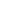Thus the Lie bracket onis given explicitly by [v, w] = [v^, w^]e.

This Lie algebrais finite-dimensional and it has the same dimension as the manifold G. The Lie algebra of G determines G up to "local isomorphism", where two Lie groups are called locally isomorphic if they look the same near the identity element.
Problems about Lie groups are often solved by first solving the corresponding problem for the Lie algebras, and the result for groups then usually follows easily.
For example, simple Lie groups are usually classified by first classifying the corresponding Lie algebras.

We could also define a Lie algebra structure on Te using right invariant vector fields instead of left invariant vector fields. This leads to the same Lie algebra, because the inverse map on G can be used to identify left invariant vector fields with right invariant vector fields, and acts as −1 on the tangent space Te.

The Lie algebra structure on Te can also be described as follows:
the commutator operation
(x, y) → xyx−1y−1

on G × G sends (e, e) to e, so its derivative yields a bilinear operation
Bilinear operator
In mathematics, a bilinear operator is a function combining elements of two vector spaces to yield an element of a third vector space that is linear in each of its arguments. Matrix multiplication is an example.-Definition:...

on TeG. This bilinear operation is actually the zero map, but the second derivative, under the proper identification of tangent spaces, yields an operation that satisfies the axioms of a Lie bracket, and it is equal to twice the one defined through left-invariant vector fields.

## Homomorphisms and isomorphisms

If G and H are Lie groups, then a Lie-group homomorphism f : G → H is a smooth group homomorphism
Group homomorphism
In mathematics, given two groups and , a group homomorphism from to is a function h : G → H such that for all u and v in G it holds that h = h \cdot h...

. (It is equivalent to require only that f be continuous rather than smooth.) The composition of two such homomorphisms is again a homomorphism, and the class of all Lie groups, together with these morphisms, forms a category
Category theory
Category theory is an area of study in mathematics that examines in an abstract way the properties of particular mathematical concepts, by formalising them as collections of objects and arrows , where these collections satisfy certain basic conditions...

. Two Lie groups are called isomorphic if there exists a bijective homomorphism between them whose inverse is also a homomorphism. Isomorphic Lie groups are essentially the same; they only differ in the notation for their elements.

Every homomorphism f : G → H of Lie groups induces a homomorphism between the corresponding Lie algebrasand. The association G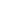is a functor
Functor
In category theory, a branch of mathematics, a functor is a special type of mapping between categories. Functors can be thought of as homomorphisms between categories, or morphisms when in the category of small categories....

(mapping between categories satisfying certain axioms).

One version of Ado's theorem
Ado's theorem
In abstract algebra, Ado's theorem states that every finite-dimensional Lie algebra L over a field K of characteristic zero can be viewed as a Lie algebra of square matrices under the commutator bracket...

is that every finite dimensional Lie algebra is isomorphic to a matrix Lie algebra. For every finite dimensional matrix Lie algebra, there is a linear group (matrix Lie group) with this algebra as its Lie algebra. So every abstract Lie algebra is the Lie algebra of some (linear) Lie group.

The global structure of a Lie group is not determined by its Lie algebra; for example, if Z is any discrete subgroup of the center of G then G and G/Z have the same Lie algebra (see the table of Lie groups
Table of Lie groups
This article gives a table of some common Lie groups and their associated Lie algebras.The following are noted: the topological properties of the group , as well as on their algebraic properties .For more examples of Lie groups and other...

for examples).
A connected Lie group is simple
Simple group
In mathematics, a simple group is a nontrivial group whose only normal subgroups are the trivial group and the group itself. A group that is not simple can be broken into two smaller groups, a normal subgroup and the quotient group, and the process can be repeated...

, semisimple, solvable
Solvable group
In mathematics, more specifically in the field of group theory, a solvable group is a group that can be constructed from abelian groups using extensions...

, nilpotent
Nilpotent group
In mathematics, more specifically in the field of group theory, a nilpotent group is a group that is "almost abelian". This idea is motivated by the fact that nilpotent groups are solvable, and for finite nilpotent groups, two elements having relatively prime orders must commute...

, or abelian
Abelian group
In abstract algebra, an abelian group, also called a commutative group, is a group in which the result of applying the group operation to two group elements does not depend on their order . Abelian groups generalize the arithmetic of addition of integers...

if and only if its Lie algebra has the corresponding property.

If we require that the Lie group be simply connected, then the global structure is determined by its Lie algebra: for every finite dimensional Lie algebraover F there is a simply connected Lie group G withas Lie algebra, unique up to isomorphism. Moreover every homomorphism between Lie algebras lifts to a unique homomorphism between the corresponding simply connected Lie groups.

## The exponential map

The exponential map
Exponential map
In differential geometry, the exponential map is a generalization of the ordinary exponential function of mathematical analysis to all differentiable manifolds with an affine connection....

from the Lie algebra Mn(R) of the general linear group
General linear group
In mathematics, the general linear group of degree n is the set of n×n invertible matrices, together with the operation of ordinary matrix multiplication. This forms a group, because the product of two invertible matrices is again invertible, and the inverse of an invertible matrix is invertible...

GLn(R) to GLn(R) is defined by the usual power series: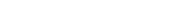for matrices A. If G is any subgroup of GLn(R), then the exponential map takes the Lie algebra of G into G, so we have an exponential map for all matrix groups.

The definition above is easy to use, but it is not defined for Lie groups that are not matrix groups, and it is not clear that the exponential map of a Lie group does not depend on its representation as a matrix group. We can solve both problems using a more abstract definition of the exponential map that works for all Lie groups, as follows.

Every vector v indetermines a linear map from R totaking 1 to v, which can be thought of as a Lie algebra homomorphism. Because R is the Lie algebra of the simply connected Lie group R, this induces a Lie group homomorphism c : R → G so that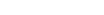for all s and t. The operation on the right hand side is the group multiplication in G. The formal similarity of this formula with the one valid for the exponential function
Exponential function
In mathematics, the exponential function is the function ex, where e is the number such that the function ex is its own derivative. The exponential function is used to model a relationship in which a constant change in the independent variable gives the same proportional change In mathematics,...

justifies the definition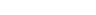This is called the exponential map
Exponential map
In differential geometry, the exponential map is a generalization of the ordinary exponential function of mathematical analysis to all differentiable manifolds with an affine connection....

, and it maps the Lie algebrainto the Lie group G. It provides a diffeomorphism
Diffeomorphism
In mathematics, a diffeomorphism is an isomorphism in the category of smooth manifolds. It is an invertible function that maps one differentiable manifold to another, such that both the function and its inverse are smooth.- Definition :...

between a neighborhood of 0 inand a neighborhood of e in G. This exponential map is a generalization of the exponential function for real numbers (because R is the Lie algebra of the Lie group of positive real numbers with multiplication), for complex numbers (because C is the Lie algebra of the Lie group of non-zero complex numbers with multiplication) and for matrices (because Mn(R) with the regular commutator is the Lie algebra of the Lie group GLn(R) of all invertible matrices).

Because the exponential map is surjective on some neighbourhood N of e, it is common to call elements of the Lie algebra infinitesimal generators of the group G. The subgroup of G generated by N is the identity component of G.

The exponential map and the Lie algebra determine the local group structure of every connected Lie group, because of the Baker–Campbell–Hausdorff formula: there exists a neighborhood U of the zero element of, such that for u, v in U we have
exp(u) exp(v) = exp(u + v + 1/2 [u, v] + 1/12 [[u, v], v] − 1/12 [[u, v], u] − ...)

where the omitted terms are known and involve Lie brackets of four or more elements. In case u and v commute, this formula reduces to the familiar exponential law exp(u) exp(v) = exp(u + v).

The exponential map from the Lie algebra to the Lie group is not always onto
Surjective function
In mathematics, a function f from a set X to a set Y is surjective , or a surjection, if every element y in Y has a corresponding element x in X so that f = y...

, even if the group is connected (though it does map onto the Lie group for connected groups that are either compact or nilpotent). For example, the exponential map of SL2(R) is not surjective. Also, exponential map is not surjective nor injective for infinite-dimensional (see below) Lie groups modelled on C Fréchet space
Fréchet space
In functional analysis and related areas of mathematics, Fréchet spaces, named after Maurice Fréchet, are special topological vector spaces. They are generalizations of Banach spaces...

, even from arbitrary small neighborhood of 0 to corresponding neighborhood of 1.

## Infinite dimensional Lie groups

Lie groups are often defined to be finite dimensional, but there are many groups that resemble Lie groups, except for being infinite dimensional. The simplest way to define infinite dimensional Lie groups is to model them on Banach space
Banach space
In mathematics, Banach spaces is the name for complete normed vector spaces, one of the central objects of study in functional analysis. A complete normed vector space is a vector space V with a norm ||·|| such that every Cauchy sequence in V has a limit in V In mathematics, Banach spaces is the...

s, and in this case much of the basic theory is similar to that of finite dimensional lie groups. However this is inadequate for many applications, because many natural examples of infinite dimensional Lie groups are not Banach manifolds. Instead one needs to define Lie groups modeled on more general locally convex topological vector spaces. In this case the relation between the Lie algebra and the Lie group becomes rather subtle, and several results about finite dimensional Lie groups no longer hold.

Some of the examples that have been studied include:
• The group of diffeomorphism
Diffeomorphism
In mathematics, a diffeomorphism is an isomorphism in the category of smooth manifolds. It is an invertible function that maps one differentiable manifold to another, such that both the function and its inverse are smooth.- Definition :...

s of a manifold. Quite a lot is known about the group of diffeomorphisms of the circle. Its Lie algebra is (more or less) the Witt algebra
Witt algebra
In mathematics, the complex Witt algebra, named after Ernst Witt, is the Lie algebra of meromorphic vector fields defined on the Riemann sphere that are holomorphic except at two fixed points. It is also the complexification of the Lie algebra of polynomial vector fields on a circle, and the Lie...

, which has a central extension called the Virasoro algebra
Virasoro algebra
In mathematics, the Virasoro algebra is a complex Lie algebra, given as a central extension of the complex polynomial vector fields on the circle, and is widely used in conformal field theory and string theory....

, used in string theory
String theory
String theory is an active research framework in particle physics that attempts to reconcile quantum mechanics and general relativity. It is a contender for a theory of everything , a manner of describing the known fundamental forces and matter in a mathematically complete system...

and conformal field theory
Conformal field theory
A conformal field theory is a quantum field theory that is invariant under conformal transformations...

. Very little is known about the diffeomorphism groups of manifolds of larger dimension. The diffeomorphism group of spacetime sometimes appears in attempts to quantize
Quantization (physics)
In physics, quantization is the process of explaining a classical understanding of physical phenomena in terms of a newer understanding known as "quantum mechanics". It is a procedure for constructing a quantum field theory starting from a classical field theory. This is a generalization of the...

gravity.
• The group of smooth maps from a manifold to a finite dimensional Lie group is an example of a gauge group (with operation of pointwise multiplication), and is used in quantum field theory
Quantum field theory
Quantum field theory provides a theoretical framework for constructing quantum mechanical models of systems classically parametrized by an infinite number of dynamical degrees of freedom, that is, fields and many-body systems. It is the natural and quantitative language of particle physics and...

and Donaldson theory
Donaldson theory
Donaldson theory is the study of smooth 4-manifolds using gauge theory. It was started by Simon Donaldson who proved Donaldson's theorem restricting the possible quadratic forms on the second cohomology group of a compact simply connected 4-manifold....

. If the manifold is a circle these are called loop group
Loop group
In mathematics, a loop group is a group of loops in a topological group G with multiplication defined pointwise. Specifically, letLG \,denote the space of continuous mapsS^1 \to G...

s, and have central extensions whose Lie algebras are (more or less) Kac–Moody algebras.
• There are infinite dimensional analogues of general linear groups, orthogonal groups, and so on. One important aspect is that these may have simpler topological properties: see for example Kuiper's theorem
Kuiper's theorem
In mathematics, Kuiper's theorem is a result on the topology of operators on an infinite-dimensional, complex Hilbert space H...

.

## See also

• Lie subgroup
Lie subgroup
In mathematics, a Lie subgroup H of a Lie group G is a Lie group that is a subset of G and such that the inclusion map from H to G is an injective immersion and group homomorphism. According to Cartan's theorem, a closed subgroup of G admits a unique smooth structure which makes it an embedded Lie...

• E8
E8 (mathematics)
In mathematics, E8 is any of several closely related exceptional simple Lie groups, linear algebraic groups or Lie algebras of dimension 248; the same notation is used for the corresponding root lattice, which has rank 8...

• Adjoint representation of a Lie group
• Adjoint endomorphism
Adjoint endomorphism
In mathematics, the adjoint endomorphism or adjoint action is an endomorphism of Lie algebras that plays a fundamental role in the development of the theory of Lie algebras and Lie groups....

• Homogeneous space
Homogeneous space
In mathematics, particularly in the theories of Lie groups, algebraic groups and topological groups, a homogeneous space for a group G is a non-empty manifold or topological space X on which G acts continuously by symmetry in a transitive way. A special case of this is when the topological group,...

• List of Lie group topics
• List of simple Lie groups
• Moufang polygon
Moufang polygon
In mathematics, a Moufang polygon, named after Ruth Moufang, is an irreducible building of rank two that admits the action of root groups.In a major book on the topic, Tits and Weiss classify them all...

• Riemannian manifold
Riemannian manifold
In Riemannian geometry and the differential geometry of surfaces, a Riemannian manifold or Riemannian space is a real differentiable manifold M in which each tangent space is equipped with an inner product g, a Riemannian metric, which varies smoothly from point to point...

• Representations of Lie groups
• Table of Lie groups
Table of Lie groups
This article gives a table of some common Lie groups and their associated Lie algebras.The following are noted: the topological properties of the group , as well as on their algebraic properties .For more examples of Lie groups and other...

The source of this article is wikipedia, the free encyclopedia.  The text of this article is licensed under the GFDL.